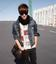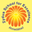Trending ▼   ResFinder# hardest paper of computer!!!

2 pages, 29 questions, 20 questions with responses, 28 total responses,00Nisarg Pandya Zydus School for Excellence (ZSE), Vejalpur, Ahmedabad
+Fave Message
 Home > cooldude0000 >Formatting page ...

Sample Paper -1 Sample Paper 1 Class X Subject COMPUTER APPLICATIONS SECTION A (All Questions are compulsory ) Q.1.a) What do you know about instance variable?  b) What does encapsulation lead to?  c) What is a Stream?  d) What is the difference in the classes String and StringBuffer?  e) What is control variable?  Q.2.a) Why is a class known as composite data type?  b) What is Recursive method?  c) What is Function Signature?  d) Differentiate a constructor from a destructor ?  e) Which unit of a class gets called when the object of the class is created ?  Q.3.a) Encapsulation wraps up the attributes and methods of the object comment.  b) Write the prototype of the function that returns a double value and takes three double parameters x, y and z as its parameters.  c) In the following statements assume a = 50 , b = 10 and c = 20. The statement is c + = ( a>0 && a<=10 ) ? + + a : a / b ; What is the value of c ?  d) A method definition is as shown below : int power ( int x , int y ) { int f = 1 ; for ( int i = 1 ; i <= y ; i + + ) f*=i; return ( f ); } Answer the following : What is the term given to the variables x and y?  What is the significance of the type int used before the method name power?  Name the type of method shown above.  In one line state that what the method shown above is computing?  i) ii) iii) iv) Q.4.a) Read the following overloaded method prototypes and answer the question that follow :  void calculate ( int x , double y ); void calculate ( double x , double y , double z ); i) Write the java statement to invoke the method calculate using actual parameters a=20 , b=45.52 . ii) Write the java prototype to overload the method calculate to return a double result And accepts three double parameters . b) Write the output of the following:  int a[ ] = { 5 , 8 , 7 , 3 , 2 }; a[ 1 ] = a[ 3 ] ; a[ 2 ] = a[ 4 ] ; a[ 3 ] = a[ 1 ] + a[ 2 ] ; for ( int i = 0 ; i < 5 ; i + + ) 1Formatting page ...